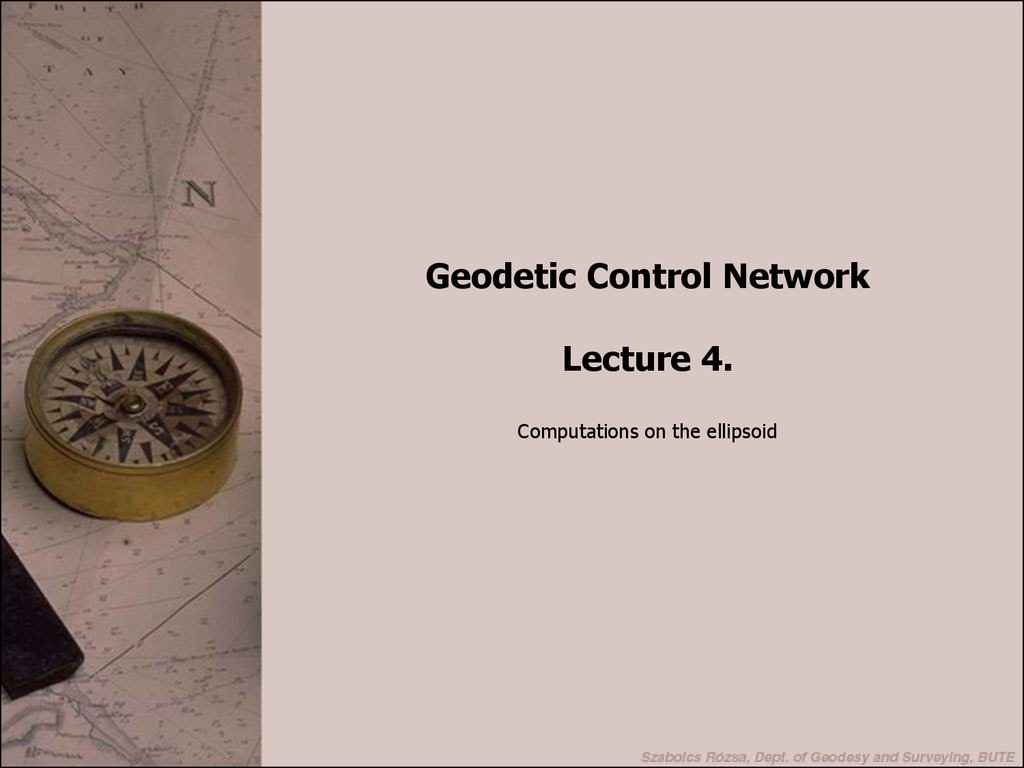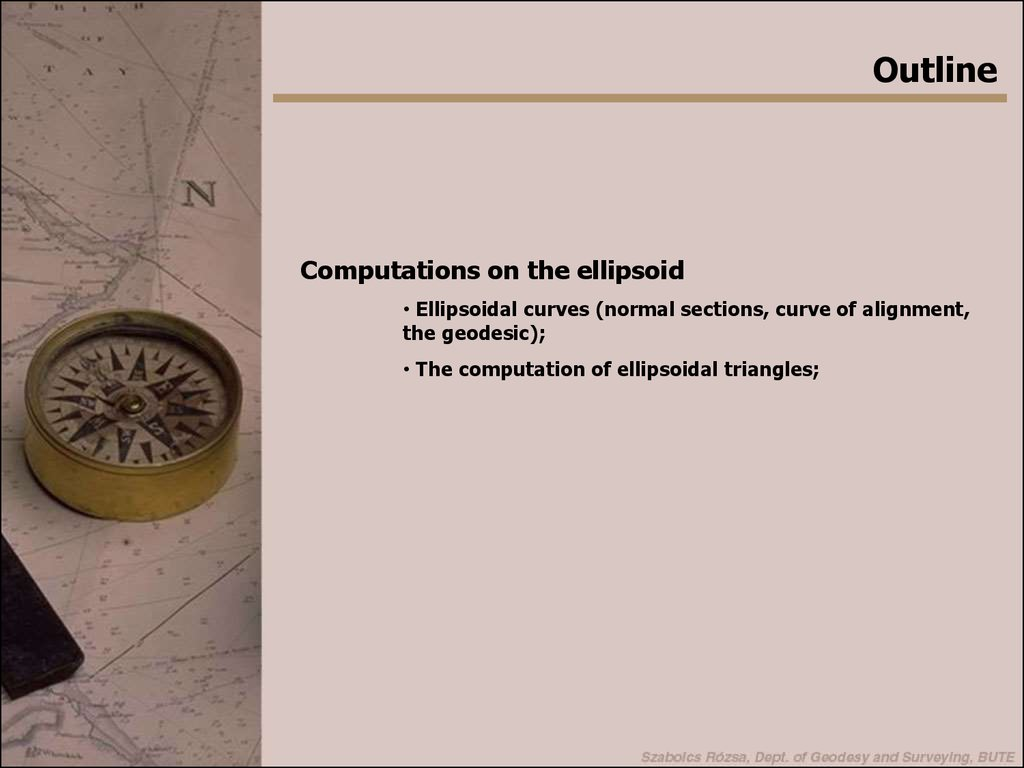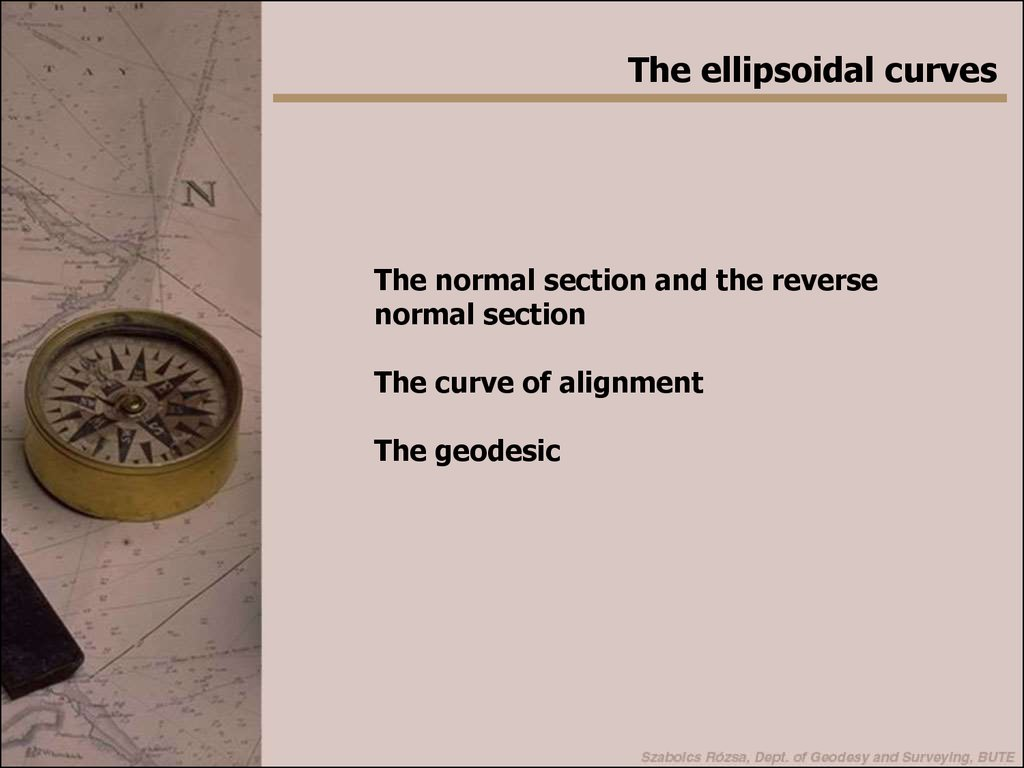# Geodetic control network. (Lecture 4)

## 1. 1. dia

Geodetic Control Network
Lecture 4.
Computations on the ellipsoid

## 2. 2. dia

Outline
Computations on the ellipsoid
• Ellipsoidal curves (normal sections, curve of alignment,
the geodesic);
• The computation of ellipsoidal triangles;

## 3. 3. dia

The ellipsoidal curves
The normal section and the reverse
normal section
The curve of alignment
The geodesic

## 4. 4. dia

The normal section
The normal section and the reverse normal section
At each ellipsoidal point an ellipsoidal normal is defined (which is
orthogonal to the surface of the ellipsoid). The intersection of those
planes, which contain the ellipsoidal normal, with the ellipsoidal
surface is called a normal section. At each point an infinite number
of normal sections exist.
Normal sections are usually ellipses. When the point is located on
the Equator, than a circular normal section can also be formed.
When a certain normal section is defined between two points on the
ellipsoid (P1P2), than it must be noted that it differs from the normal
section between P2P1, since the ellipsoidal normals have a
skewness. The latter section is called the reverse normal section.
P2
P1
n
tio
se
c
a
al
rm
n
tio
no
rm
rse
ve
Re
no
c
l se

## 5. 5. dia

The normal section
The normal section and the reverse normal section has an
angle :
1
s2 2
' ' 2 e cos2 1 sin 2
4
a
The maximal distance between the normal section and the
reverse normal section is:
1 s3 2
2
d
e
cos
1 sin 2
2
16 N1
Where N1 is the radius of the curvature in the prime vertical.

## 6. 6. dia

The normal section
Notes on the normal section:
• when both of the points are located on the same meridian, then
the normal section and the reverse normal section coincide.
• when both of the points are located on the Equator, than both
the normal and the reverse normal section coincides with the
Equator.
• when both of the points are located on the same parallel curve
(same latitude), then the normal section lies not on the parallel
curve, but on the opposite side of the equator.

## 7. 7. dia

Disadvantage of the normal section
• When we want measure the angles of a triangle and connect the
nodes of the triangle with normal sections, then the observed angles
are not consequent. -> a different ellipsoidal curve should be used
for the representation

## 8. 8. dia

The curve of alignment
It is usually used in the Anglo-Saxon
region.
Let’s suppose that P1 and P2 are two ellipsoidal
points. Let’s connect the P1 and P2 and form a
chord inside the ellipsoid. By drawing the
ellipsoidal normal from each point of the P1P2 chord, the
intersections of these normals form the curve of alignment.
When in any point of the CoA an ellipsoidal normal is drawn, and a
vertical plane is created, which contains P1, then P2 lies in this
vertical plane as well, since the plane contains two points of the
chord, thus it must contain all the points on the chord.
Thus the CoA can be defined as the sum of those points, in
which the normal sections pointing to P1 and P2 has the
azimuth difference of 180°.
The CoA connects to the normal sections of P1 and P2 tangential ->
this is the main advantage, since the angular observations are equal
to the angles between the curves of alignment.

## 9. 9. dia

The curve of alignment
Notes on the CoA:
• When P1 and P2 are on the same meridian, then the CoA is the
meridian itself;
• When P1 and P2 are on the Equator, then the CoA is the Equator.
• When P1 and P2 are on the same parallel curve, then the CoA is
located from both the parallel curve and the normal section in the
opposite side of the Equator.

## 10. 10. dia

The geodesic
The general solution to define the sides of the ellipsoidal triangles is
application of the geodesic.
To define the geodesic, first we need to brush up our knowledge on the
Frenet trihedron.
For any points on any curve in the 3D space three mutually orthogonal
vectors can be created (the Frenet trihedron), which are:
• the tangent;
• the principal normal (perpendicular
to the tangent, and is aligned with
the radius of curvature of the curve;
• the binormal (perpendicular to both
the tangent and the principal normal;

## 11. 11. dia

The geodesic
The Frenet-frame contains three different planes (formed by a pair
of the three vectors):
• normal plane (the plane of the principal normal and the binormal);
• osculating plane (the plane of the principal normal and the tangent);
the princ ipal normal
• rectifying plane (the plane of the tangent and the binormal).
tangent
the
o rm
b in
al

## 12. 12. dia

The geodesic
The geodesic: is an ellipsoidal curve, where at each point of the
curve the principal normal of the curve conincides with the normal of
the ellipsoid.
Or: In each point the osculating plane of the curve is a normal plane
of the ellipsoidal surface.
Or: The rectifying plane of the curve coincides the tangential plane
of the ellipsoidal surface.
Or: The geodesic is a specific curve among the curves on the
surface, that has the shortest path between
the two points. This is a sufficient criteria,
but NOT a required one (helix against the
A
straight line between two points on the same
element of a cylinder).
Example:
• straight lines on the surfaces are geodesics
(e.g. cylinder)
B

## 13. 13. dia

Graphical derivation of geodesic on the ell.

## 14. 14. dia

Properties of the geodesic
se
er
v
re
rm
no
a
t
ec
s
l
rm
no
a
the geodesic
n
io
t
ec
s
l
n
io

## 15. 15. dia

The geodesic on surfaces of rotation
On surfaces of rotation the following equation can be derived
for the geodesic (Clairaut-equation):
r sin C
Where
r is the radius of the paralel circle;
is the azimuth of the curve at the point;
C is constant.
A required but not sufficient criteria!

## 16. 16. dia

The solution of ellipsoidal triangles
Solution: the computation of the length of the sides of the
triangle from angular observations and one distance
observation!
The ellipsoid is usually approximated by a sphere.
When the study area is relatively small, then the Gauss-theorem is
used, which states that an infinitesimal part of the ellipsoid can be
approximated by an infinitesimal part of a sphere. The radius of
such a sphere is the mean-radius of the ellipsoid in the centroid of
the infinitesimal part:
R MN
where
M is the curvature in the meridian direction
N is the curvature of the prime vertical

## 17. 17. dia

The excess angle of the ellipsoidal triangle
Ellipsoidal triangles are approximated by sperical tirangles.
The spherical excess angle:
'' ''
F
0,005F
2
R
When the triangles are small (planar approximation):
1
F bc sin
2
Where b, c are the sides of the triangle and is the angle between
them.
Since (sine-theorem)
c sin
b
sin

## 18. 18. dia

The excess angle of the ellipsoidal triangle
The excess angle:
F
bc sin
c 2 sin sin
'' '' 2 ''
''
2
R
2R
2R 2 sin
In case of large triangles (bigger than 5000 km2) the ellipsoidal
excess angle should be computed:
F a 2 b2 c 2
' ' ' ' 2 1
2
R
24R

## 19. 19. dia

The Legendre method
The ellipsodal triangles are approximated by spherical triangles
(Gauss-theorem) that has the same angles as the ellipsoidal ones.
Legendre prooved that unless the triangle is big, the triangles can
be solved with the planar approximation, when the spherical angles
are decreased by one third of the excess angle.
'
3
'
'
3
3
a' a
b' b
c' c

## 20. 20. dia

The Legendre method
When the spherical (ellipsoidal) triangle is not small enough, then
m2 a 2
' 1
2
3
20 R
m2 b2
' 1
3
20 R 2
m2 c2
' 1
2
3
20 R
where
a2 b2 c2
m
3
2
Note: the difference reaches the level of 0.001” in the
distance of 200km only!

## 21. 21. dia

The Soldner method
a3
a' a 2
6R
b3
b' b 2
6R
c3
c' c 2
6R
'
'
'

## 22. 22. dia

The computation of coordinates on the ell.
1st and 2nd fundamental task
• solving polar ellipsoidal triangles
• P1P2 curve is usually a geodesic, in English literature mostly
the normal section is used and the results are corrected for the
geodesic

## 23. 23. dia

The computation of coordinates on the ell.
Various solution depending on the distance:
• up to 200 km (polynomial solutions)
• up to 1000 km (usually based on normal sections) used by long
baseline distance observations
• up to 20000 km (air navigation) based on the Clairaut equation
We’ll focus on the first one!

## 24. 24. dia

Legendre’s polynomial method
Known P1 ( 1,l1) and 1,2, then the 2,l2 coordinates and the 2,1
azimuth depends on the ellipsoidal distance only.
2 f1 s
l 2 f 2 s
2,1 f 3 s
These function can be written in MacLaurin series:
2 f1 s 2 3 f1 s 3
f1
2 f1 s 0 s 2
3 ...
s
s
2
0
0
s 6
2 f2 s 2 3 f2 s3
f 2
l2 f 2 s 0 s 2
3 ...
s 0 s 0 2 s 6
2 f3 s 2 3 f3 s3
f 3
2,1 180 f 3 s s 2
3 ...
s 0 s 0 2 s 6

## 25. 25. dia

Legendre’s polynomial method
2 f1 s 2 3 f1 s 3
f1
2 f1 s 0 s 2
3 ...
s 0 s 0 2 s 6
2 f2 s 2 3 f2 s3
f 2
l2 f 2 s 0 s 2
3 ...
s 0 s 0 2 s 6
2 f3 s 2 3 f3 s3
f 3
2,1 180 f 3 s 0 s 2
3 ...
s
s
2
0
0
s 6
f1 s 0 1
f1
d
cos
f 2 s 0 l1
s
ds
M
f 3 s 0 12
f 2
dl
sin
s
ds
N cos
f 3
d
sin tan
s
ds
N
The differential equation system
of the geodesic

## 26. 26. dia

Legendre’s polynomial method
Practical computations using the Legendre’s method:
• slow convergence of the series (s=100, n=5; s=30, n=4);
• Schoeps (1960) published tables containing the polynomial
coefficients depending on the position;
• Gerstbach (1974) published computational formulae for calculators
and computers (s<60km, 45° < < 54.5°, accuracy is 0.0001”)
Gerstbach formulae will be
used during the practicals!

## 27. 27. dia

Gauss’s method of mean latitudes
Principle: The Legendre series are applied to the
middle of the geodesic -> shorter arc -> faster
convergence -> n is less
Problem: 0, l0, 0 is not known, since 2, l2, 21 is
the purpose of the computations! Iterative
computations!

## 28. 28. dia

Gauss’s method of mean latitudes
Now it is a useful method, since the coordinates of both endpoints
are known.
Writing the Legendre polynomials for P1:
2
3
f1 s 1 f1 s 1 f1 s
1 f1 s 0 2 3 ...
s 0 2 2 s 0 2 6 s 2
2
3
2
3
f 2 s 1 f 2 s 1 f 2 s
l1 f 2 s 0 2 3 ...
s 0 2 2 s 0 2 6 s 2
2
3
2
f 3 s 1 f 3 s
f 3 s 0
2
s
0 2 2 s 0 2
2
1, 2
1 3 f 3 s
3 ...
6 s 2
3

## 29. 29. dia

Writing the Legendre polynomials for P2:
2
3
f1 s 1 f1 s 1 f1 s
2 f1 s 0 2 3 ...
s 0 2 2 s 0 2 6 s 2
2
3
2
3
f 2 s 1 f 2 s 1 f 2 s
l2 f 2 s 0 2 3 ...
s 0 2 2 s 0 2 6 s 2
2
3
2
f 3 s 1 f 3 s
2,1 180 f 3 s 0 2
s 0 2 2 s 0 2
2
1 3 f 3 s
3 ...
6 s 2
3
Where:
f1 s 0 0
f 2 s 0 l0
f 3 s 0 01 180 02 0
0
1 2
2

## 30. 30. dia

Let’s compute the difference of the Legendre polynomials between
P2 and P1:
2 1
l l2 l1
20 10 180 21 12 180
1 3 f1 3
f1
s 3 s ...
24 s 0
s 0
1 3 f2 3
f 2
l
s 3 s ...
24 s 0
s 0
1 3 f3 3
f 3
s 3 s ...
24 s 0
s 0

## 31. 31. dia

1 3 f1 3
f1
s 3 s ...
24 s 0
s 0
1 3 f2 3
f 2
l
s 3 s ...
24 s 0
s 0
1 3 f3 3
f 3
s 3 s ...
24 s 0
s 0
The first two equations can be solved for:
s cos 0 X
s sin 0 Y
Dividing the two results 0 can be computed:
Y
, and
X
X
Y
s
cos 0 sin 0
tan 0